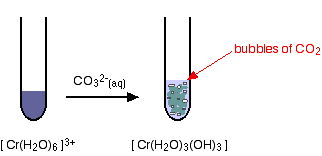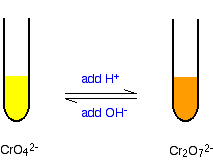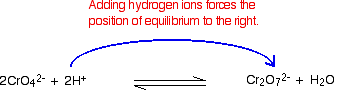Chemistry of Chromium

•• Contributed by Jim Clark
• Former Head of Chemistry and Head of Science at Truro School in Cornwall

This page looks at some aspects of chromium chemistry. It includes: reactions of chromium(III) ions in solution (summarised from elsewhere on the site); the interconversion of the various oxidation states of chromium; the chromate(VI)-dichromate(VI) equilibrium; and the use of dichromate(VI) ions as an oxidizing agent (including titrations).

Reactions of chromium(III) ions in solution

The simplest ion that chromium forms in solution is the hexaaquachromium(III) ion - [Cr(H2O)6]3+.

The acidity of the hexaaqua ions

In common with the other 3+ ions, the hexaaquachromium(III) ion is fairly acidic - with a pH for typical solutions in the 2 - 3 range.

The ion reacts with water molecules in the solution. A hydrogen ion is lost from one of the ligand water molecules:

$\ce{Cr(H2O)_6^{3+} + H2O <=> Cr(H2O)5(OH)^{2+} + H3O^{+}}$

The complex ion is acting as an acid by donating a hydrogen ion to water molecules in the solution. The water is, of course, acting as a base by accepting the hydrogen ion. Because of the confusing presence of water from two different sources (the ligands and the solution), it is easier to simplify this:

$\ce{Cr(H2O)_6^{3+} <=> Cr(H2O)5(OH)^{2+} + H^{+} (aq)}$

However, if you write it like this, remember that the hydrogen ion isn't just falling off the complex ion. It is being pulled off by a water molecule in the solution. Whenever you write "H+(aq)" what you really mean is a hydroxonium ion, H3O+.

Ligand exchange reactions involving chloride or sulfate ions

The hexaaquachromium(III) ion is a "difficult to describe" violet-blue-grey color. However, when it is produced during a reaction in a test tube, it is often green. We nearly always describe the green ion as being Cr3+(aq) - implying the hexaaquachromium(III) ion. That's actually an over-simplification. What happens is that one or more of the ligand water molecules get replaced by a negative ion in the solution - typically sulfate or chloride.

Replacement of the water by sulfate ions

You can do this simply by warming some chromium(III) sulfate solution.One of the water molecules is replaced by a sulfate ion. Notice the change in the charge on the ion. Two of the positive charges are canceled by the presence of the two negative charges on the sulfate ion.

Replacement of the water by chloride ions

In the presence of chloride ions (for example with chromium(III) chloride), the most commonly observed color is green. This happens when two of the water molecules are replaced by chloride ions to give the tetraaquadichlorochromium(III) ion - [Cr(H2O)4Cl2]+. Once again, notice that replacing water molecules by chloride ions changes the charge on the ion.

Reactions of hexaaquachromium(III) ions with hydroxide ions

Hydroxide ions (from, say, sodium hydroxide solution) remove hydrogen ions from the water ligands attached to the chromium ion.

Once a hydrogen ion has been removed from three of the water molecules, you are left with a complex with no charge - a neutral complex. This is insoluble in water and a precipitate is formed.

$\ce{Cr(H2O)_6^{3+} + 3OH^{-} -> [Cr(H2O)3(OH)3] (s) + 3H2O}$

But the process doesn't stop there. More hydrogen ions are removed to give ions like $$\ce{[Cr(H2O)2(OH)4]^{-}}$$ and $$\ce{[Cr(OH)6]^{3-}}$$. For example:

$\ce{[Cr(H2O)3(OH)3] (s) + 3OH^{-} -> [Cr(OH)6]^{3-} (aq) + 3H2O}$

The precipitate redissolves because these ions are soluble in water. In the test-tube, the color changes are:Reactions of hexaaquachromium(III) ions with ammonia solution

The ammonia acts as both a base and a ligand. With a small amount of ammonia, hydrogen ions are pulled off the hexaaqua ion exactly as in the hydroxide ion case to give the same neutral complex.

$\ce{[Cr(H2O)6]^{3+} (aq) + 3NH3 (aq) -> [Cr(H2O)3(OH)3] (s) + 3NH4^{+} (aq)}$

That precipitate dissolves to some extent if you add an excess of ammonia (especially if it is concentrated). The ammonia replaces water as a ligand to give hexaamminechromium(III) ions (this is an example of a ligand exchange reaction).

$\ce{[Cr(H2O)6]^{3+} (aq) + 6NH3 (aq) -> [Cr(NH3)6]^{3+} (aq) + 6 H2O (l)}$

The color changes are:Reactions of hexaaquachromium(III) ions with carbonate ions

If you add sodium carbonate solution to a solution of hexaaquachromium(III) ions, you get exactly the same precipitate as if you added sodium hydroxide solution or ammonia solution. This time, it is the carbonate ions which remove hydrogen ions from the hexaaqua ion and produce the neutral complex.

Depending on the proportions of carbonate ions to hexaaqua ions, you will get either hydrogencarbonate ions formed or carbon dioxide gas from the reaction between the hydrogen ions and carbonate ions. The more usually quoted equation shows the formation of carbon dioxide.

$\ce{2[Cr(H2O)6]^{3+} (aq) + 3CO3^{2-} (aq) -> 2[Cr(H2O)3(OH)3] (s) + 3 CO2 (g) + 3H2O (l)}$

Apart from the carbon dioxide, there is nothing new in this reaction:The oxidation of chromium(III) to chromium(VI)

An excess of sodium hydroxide solution is added to a solution of the hexaaquachromium(III) ions to produce a solution of green hexahydroxochromate(III) ions.This is then oxidised by warming it with hydrogen peroxide solution. You eventually get a bright yellow solution containing chromate(VI) ions.The equation for the oxidation stage is:

$\ce{2[Cr(OH)6]^{3-} (aq) + 3H2O2 (aq) -> 2CrO4^{2-} + 2OH^{-} + 8H2O (l)}$

Some chromium(VI) chemistry

The chromate(VI)-dichromate(VI) equilibrium

You are probably more familiar with the orange dichromate(VI) ion, $$\ce{Cr2O7^{2-}}$$, than the yellow chromate(VI) ion, $$\ce{CrO4^{2-}}$$. Changing between them is easy; i f dilute sulfuric acid is added to the yellow solution it turns orange. If you add sodium hydroxide solution to the orange solution it turns yellow.The equilibrium reaction at the heart of the interconversion is:

$\ce{2CrO_4^{2-} + 2H^+ <=> Cr_2O_7^{2-} + H_2O}$

If you add extra hydrogen ions to this, the equilibrium shifts to the right, which is consistent with Le Chatelier's Principle.If you add hydroxide ions, these react with the hydrogen ions. The equilibrium tips to the left to replace them.This equilibration is also disturbed by adding base too.Figure $$\PageIndex{1}$$: Equilibrium between chromate and dichromate. By adding $$NaOH$$, the equilibrium shifts to the right (to the chromate), and the solution is yellow. Figure used with permission from Wikipedia.

Making potassium dichromate(VI) crystals

Potassium dichromate crystals can be made by a combination of the reactions we've already looked at on this page.

Starting from a source of chromium(III) ions such as chromium(III) chloride solution:

You add potassium hydroxide solution to give first a grey-green precipitate and then the dark green solution containing [Cr(OH)6]3- ions. This is all described in detail further up the page. Notice that you have to use potassium hydroxide. If you used sodium hydroxide, you would end up eventually with sodium dichromate(VI).

Now you oxidize this solution by warming it with hydrogen peroxide solution. The solution turns yellow as potassium chromate(VI) is formed. This reaction is also described further up the page.

All that is left is to convert the yellow potassium chromate(VI) solution into orange potassium dichromate(VI) solution. You may remember that that is done by adding acid. This is described above if you have forgotten.

Unfortunately there is a problem here. Potassium dichromate will react with any excess hydrogen peroxide to give initially an unstable deep blue solution and it eventually gives the original chromium(III) ions again! To get around this, you first need to destroy any excess hydrogen peroxide.

This is done by boiling the solution. Hydrogen peroxide decomposes on heating to give water and oxygen. The solution is boiled until no more bubbles of oxygen are produced. The solution is heated further to concentrate it, and then concentrated ethanoic acid is added to acidify it. Orange crystals of potassium dichromate are formed on cooling.

The reduction of dichromate(VI) ions with zinc and an acid

Dichromate(VI) ions (for example, in potassium dichromate(VI) solution) can be reduced to chromium(III) ions and then to chromium(II) ions using zinc and either dilute sulfuric acid or hydrochloric acid.

Hydrogen is produced from a side reaction between the zinc and acid. This must be allowed to escape, but you need to keep air out of the reaction. Oxygen in the air rapidly re-oxidises chromium(II) to chromium(III).

An easy way of doing this is to put a bit of cotton wool in the top of the flask (or test-tube) that you are using. This allows the hydrogen to escape, but stops most of the air getting in against the flow of the hydrogen.The reason for the inverted commas around the chromium(III) ion is that this is a simplification. The exact nature of the complex ion will depend on which acid you use in the reduction process. This has already been discussed towards the top of the page.

Using potassium dichromate(VI) as an oxidizing agent in organic chemistry

Potassium dichromate(VI) solution acidified with dilute sulfuric acid is commonly used as an oxidising agent in organic chemistry. It is a reasonably strong oxidising agent without being so powerful that it takes the whole of the organic molecule to pieces! (Potassium manganate(VII) solution has some tendency to do that.)

It is used to:

• oxidize secondary alcohols to ketones;
• oxidize primary alcohols to aldehydes;
• oxidize primary alcohols to carboxylic acids.

For example, with ethanol (a primary alcohol), you can get either ethanal (an aldehyde) or ethanoic acid (a carboxylic acid) depending on the conditions.

• If the alcohol is in excess, and you distil off the aldehyde as soon as it is formed, you get ethanal as the main product. $\ce{Cr2O7^{2-} + 8H^{+} + 3CH3CH2OH \rightarrow 2Cr^{3+} + 7H2O + 3CH3CHO} \label{ox1}$

• If the oxidizing agent is in excess, and you do not allow the product to escape -e.g., by heating the mixture under reflux (heating the flask with a condenser placed vertically in the neck) - you get ethanoic acid. $\ce{2Cr2O7^{2-} + 16H^{+} + 3CH3CH2OH \rightarrow 4Cr^{3+} + 11H2O + 3CH3COOH}$

These equations are often simplified to concentrate on what is happening to the organic molecules. For example, the last two could be written:

$\ce{CH3CH2OH + [O] \rightarrow CH3CHO + H2O}$

$\ce{CH3CH2OH + 2[O] \rightarrow CH3COOH + H2O}$

The oxygen written in square brackets just means "oxygen from an oxidizing agent".

Using this same reaction to make chrome alum crystals

You will find chrome alum under all sorts of different names:

• chrome alum
• potassium chromium(III) sulfate
• chromium(III) potassium sulfate
• chromium(III) potassium sulfate-12-water
• chromium(III) potassium sulfate dodecahydrate

. . . and various others!

You will also find variations on its formula. For example:

• CrK(SO4)2,12H2O
• Cr2(SO4)3,K2SO4,24H2O
• K2SO4,Cr2(SO4)3,24H2O

The first of these formulae is just the other ones divided by two and rearranged a bit although the second one is easier to understand what is going on.

Chrome alum is known as a double salt. If you mix solutions of potassium sulfate and chromium(III) sulfate so that their molar concentrations are the same, the solution behaves just like you would expect of such a mixture. It gives the reactions of chromium(III) ions, of potassium ions, and of sulfate ions.

However, if you crystallise it, instead of getting mixed crystals of potassium sulfate and chromium(III) sulfate, the solution crystallizes as single deep purple crystals. These are "chrome alum". Chrome alum crystals can be made by reducing acidified potassium dichromate(VI) solution using ethanol, and then crystallizing the resulting solution. Assuming you use an excess of ethanol, the main organic product will be ethanal - and we've already seen this before (Equation \ref{ox1}):

$\ce{Cr2O7^{2-} + 8H^{+} + 3CH3CH2OH \rightarrow 2Cr^{3+} + 7H2O + 3CH3CHO} \nonumber$

This ionic equation obviously does not contain the spectator ions, potassium and sulfate. Feeding those back in gives the full equation:

$\ce{K2Cr2O7 + 4HSO4 + 3CH3CH2OH \rightarrow Cr2(SO4)3 + K2SO4 + 7H2O + 3CH3CHO}$

You will see that the chromium(III) sulfate and potassium sulfate are produced in exactly the right proportions to make the double salt.

In the Lab

What you do, then, is this:

You start with a solution of potassium dichromate(VI) to which has been added some concentrated sulfuric acid. The solution is then cooled by standing it in ice. An excess of ethanol is added slowly with stirring so that the temperature doesn't rise too much. When all the ethanol has been added, the solution is left over-night, preferably in a refrigerator, to crystallize. The crystals can be separated from the remaining solution, washed with a little pure water and then dried with filter paper.

Using potassium dichromate(VI) as an oxidising agent in titrations

Potassium dichromate(VI) is often used to estimate the concentration of iron(II) ions in solution. It serves as an alternative to using potassium manganate(VII) solution.

In practice

There are advantages and disadvantages in using potassium dichromate(VI).

• Potassium dichromate(VI) can be used as a primary standard. That means that it can be made up to give a stable solution of accurately known concentration. That isn't true of potassium manganate(VII).

• Potassium dichromate(VI) can be used in the presence of chloride ions (as long as the chloride ions aren't present in very high concentration).

Potassium manganate(VII) oxidises chloride ions to chlorine; potassium dichromate(VI) isn't quite a strong enough oxidising agent to do this. That means that you don't get unwanted side reactions with the potassium dichromate(VI) soution.

• The main disadvantage lies in the color change. Potassium manganate(VII) titrations are self-indicating. As you run the potassium manganate(VII) solution into the reaction, the solution becomes colorless. As soon as you add as much as one drop too much, the solution becomes pink - and you know you have reached the end point.

Unfortunately potassium dichromate(VI) solution turns green as you run it into the reaction, and there is no way you could possibly detect the color change when you have one drop of excess orange solution in a strongly colored green solution.

With potassium dichromate(VI) solution you have to use a separate indicator, known as a redox indicator. These change color in the presence of an oxidising agent.

There are several such indicators - such as diphenylamine sulfonate. This gives a violet-blue color in the presence of excess potassium dichromate(VI) solution. However, the color is made difficult by the strong green also present. The end point of a potassium dichromate(VI) titration isn't as easy to see as the end point of a potassium manganate(VII) one.

The calculation

The half-equation for the dichromate(VI) ion is:

$\ce{Cr2O7^{2-} + 14H^{+} + 6e^{-} -> 2Cr^{3+} + 7H2O}$

. . . and for the iron(II) ions is:

$\ce{Fe^{2+} \rightarrow Fe^{3+} + e^{-}}$

Combining these gives:

$\ce{Cr2O7^{2-} + 6 Fe^{2+} + 14H^{+} + 6e^{-} -> 2Cr^{3+} + 6 Fe^{3+} + 7H2O}$

You can see that the reacting proportions are 1 mole of dichromate(VI) ions to 6 moles of iron(II) ions. Once you have established that, the titration calculation is going to be just like any other one.

Testing for chromate(VI) ions in solution

Typically, you would be looking at solutions containing sodium, potassium or ammonium chromate(VI). Most chromates are at best only slightly soluble; many we would count as insoluble. The bright yellow color of a solution suggests that it would be worth testing for chromate(VI) ions.

Testing by adding an acid

If you add some dilute sulfuric acid to a solution containing chromate(VI) ions, the color changes to the familiar orange of dichromate(VI) ions.You can't rely on this as a test for chromate(VI) ions, however. It might be that you have a solution containing an acid-base indicator which happens to have the same color change!

Testing by adding barium chloride (or nitrate) solution

Chromate(VI) ions will give a yellow precipitate of barium chromate(VI).

$\ce{Ba^{2+} (aq) + CrO4^{2+}(aq) \rightarrow BaCrO4(s)}$$\ce{Pb^{2+} (aq) + CrO4^{2-} (aq) \rightarrow PbCrO4(s) }$